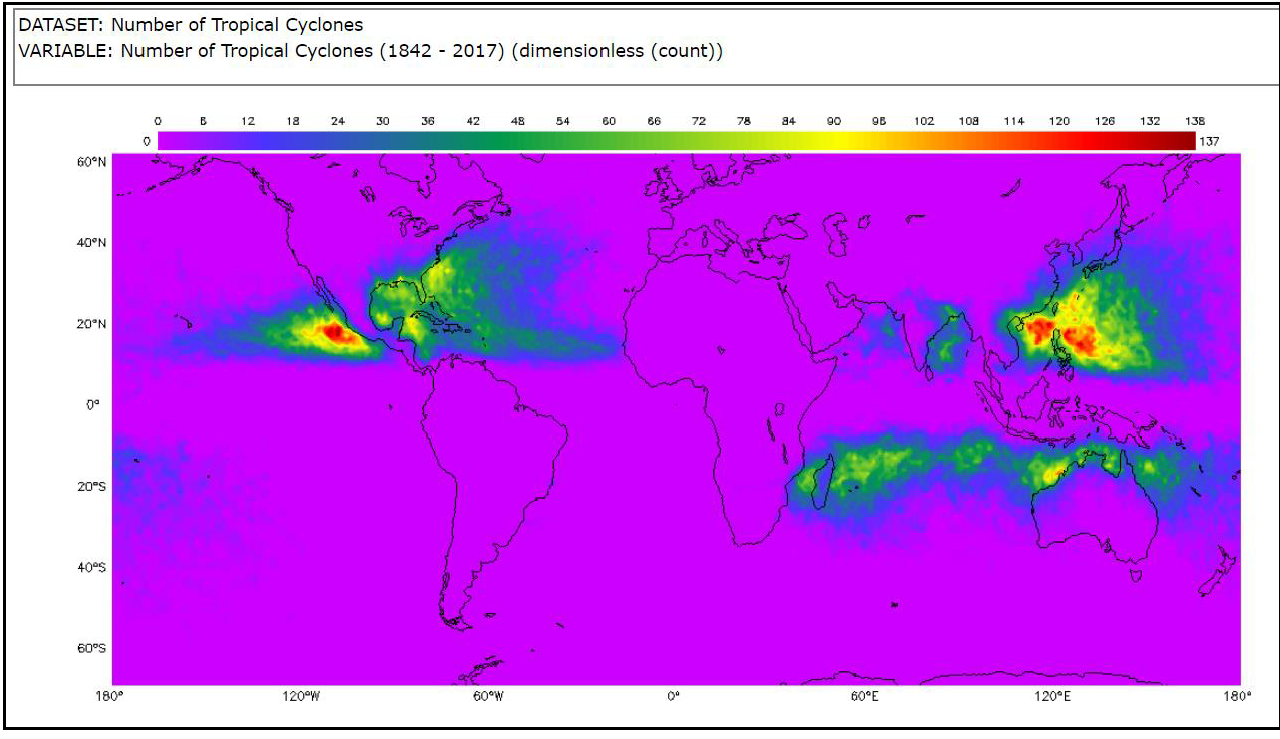## Tropical Cyclone Counts Box Plot

Students will analyze and interpret a box plot and evaluate the spread of the data. Students will compare it with a different visualization of the data to see how the two compare. Students will discuss the limitations of the two types of data displays and formulate questions to help them develop data literacy skills. They can create the box plot, or use the one provided. This is part of the Tropical Cyclone Counts Graphing Bundle and can be completed independently or with the other activities in the bundle.

### Mini Lesson

Introduce and post the driving question:

Which data display is most useful for determining the risk of a tropical cyclone in a given area and preparing an effective emergency plan?

### Analyze Tropical Cyclone Map Image

If students already completed the Tropical Cyclone Counts Histogram or the Tropical Cyclone Counts Scatter Plot mini lesson, quickly review the patterns they identified, use the maps that were previously marked and continue with the Review Box Plots section.

Tropical cyclones are sometimes called hurricanes or typhoons. The image is a model of the number of tropical cyclones around the world from 1842 – 2017. It was generated in the My NASA Data Earth System Data Explorer.

####### Think-Pair-Share

1. Examine the map at 120° W. What do you see at the different latitudes?

2. Circle the area on the map at 120° W with the most tropical cyclones.

3. Put a box around the areas on the map at 120° W between the equator and 40° N with the lowest number of tropical cyclones.

4. Describe the patterns you see.

Have students share what they discussed.

#### Review Box Plots

Option 1: Have students complete the Tropical Cyclone Counts Box Plot Graphing Activity to make a box plot of the data for the number of tropical cyclones at 120° W for each full degree of latitude from the equator to 40° N. This will be used for the rest of this lesson and can also be used in Mini Lesson 5 or the Compare Tropical Cyclone Groups Lesson.

Option 2: Use the box plot provided for the number of tropical cyclones at 120° W for each full degree of latitude from the equator to 40° N.

• A box plot is a type of graph which shows variability within a group. It shows the maximum, minimum, median, upper and lower quartile of the data.
• It also shows the spread of the data. Each section of the plot shows 25% of the data.
• The types of questions that might be answered with a box plot include:
• What is the range of the populations of different countries?
• What is the test score in a class?
• What is the range for the top 25% of the scores on a test? The bottom 25%?
• What is the most/least chocolate chips the bags sampled?
• How large is the spread of the data?
• Box plots are very useful for comparing data sets to each other.
• Box plot are most often displayed horizontally. Box plots created in software programs are sometimes vertical. Either representation is valid.

#### Question Set 1

Group students and have them interpret the box plot using Question Set 1

1. What does the box plot show?

2. What is unique about this box plot?

3. What is the overall distribution of the box plot?

4. What does the shape of the distribution tell you?

5. What does it NOT show?

6. What are the maximum, minimum, range, median, first quartile, third quartile, and interquartile range?

#### Question Set 2

1. How do the map and box plot show the total number of tropical cyclones in the region?

2. The number of tropical cyclones at each latitude can be found on __________

3. The quartile in which a given number of tropical cyclones falls can be seen on the _______.

4. The pattern in the map shows ________ while the pattern in the box plot shows _______.

5. What kind of questions can you answer with a box plot?

6. What do you wonder from the box plot? Can you answer it with this graph, or do you need to see the data in a different way?
7. Does the box plot help you answer the driving question “Which data display is most useful for determining the risk of a tropical cyclone in a given area and preparing an effective emergency plan?”

#### Exit Ticket

Have students prepare an exit ticket to write a caption for the box plot using the I2 technique?

• What I see…
• What it means…
• Write a caption for the box plot.

Collect exit tickets.